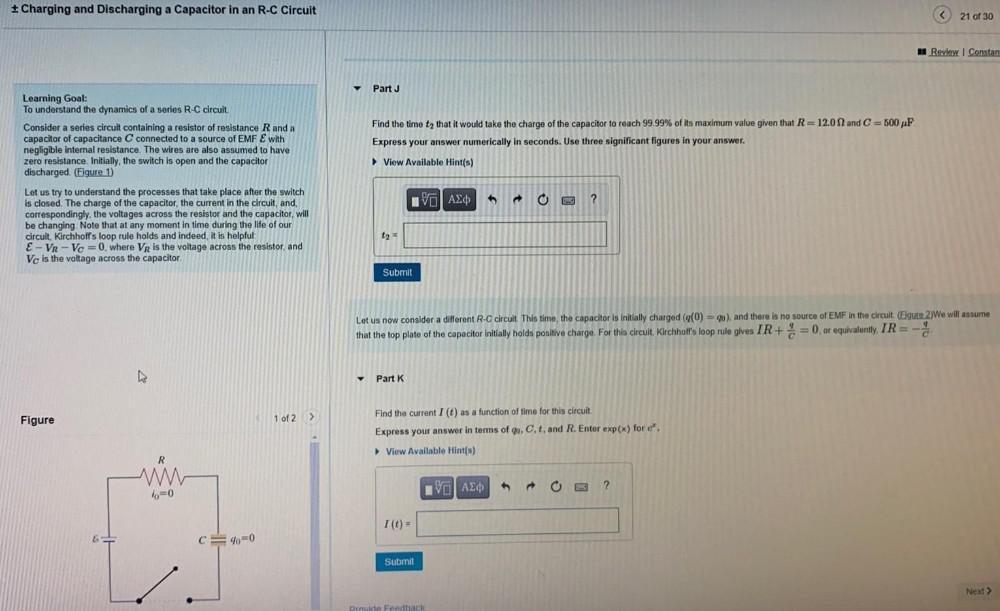Question:

# + Charging and Discharging a Capacitor in an R-C Circuit < 21 of 30 Review Constan y Part J Learning Goal: To understand the dyn+ Charging and Discharging a Capacitor in an R-C Circuit < 21 of 30 Review Constan y Part J Learning Goal: To understand the dynamics of a series R-C circuit. Consider a series circuit containing a resistor of resistance R and a capacitor of capacitance C connected to a source of EMF & with negligible internal resistance. The wires are also assumed to have zero resistance. Initially, the switch is open and the capacitor discharged. (Figure 1) Find the time ty that it would take the charge of the capacitor to reach 99.99% of its maximum value given that R=12.0 N and C = 500 F Express your answer numerically in seconds. Use three significant figures in your answer. ► View Available Hint(s) IVO AO ? Let us try to understand the processes that take place after the switch is closed. The charge of the capacitor, the current in the circuit, and, correspondingly, the voltages across the resistor and the capacitor, will be changing. Note that at any moment in time during the life of our circuit, Kirchhoff's loop rule holds and indeed, it is helpful E - VR-Vc =0, where VR is the voltage across the resistor, and Vc is the voltage across the capacitor t2 = Submit 9 Let us now consider a different R-C circuit. This time, the capacitor is initially charged (g(0) = qo), and there is no source of EMF in the circuit (Eigure 2)We will assume that the top plate of the capacitor initially holds positive charge. For this circuit, Kirchhoff's loop rule gives IR+ 8 = 0, or equivalently, IR = Part K Figure 1 of 2 > Find the current I (t) as a function of time for this circuit. Express your answer in terms of go, C, t, and R. Enter exp(x) for c. View Available Hint(s) R VALO ? 10=0 1 (t) = C=90=0 Submit J Next > Provide Feedback